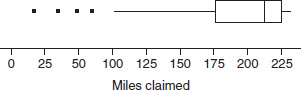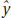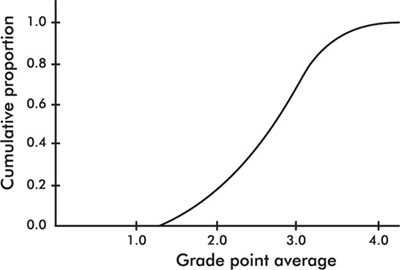# AP Statistics Multiple-Choice Practice Test 27

### Test Information10 questions23 minutes

1. Which of the following is a binomial random variable?

2. Company I manufactures demolition fuses that burn an average of 50 minutes with a standard deviation of 10 minutes, while company II advertises fuses that burn an average of 55 minutes with a standard deviation of 5 minutes. Which company's fuse is more likely to last at least 1 hour? Assume normal distributions of fuse times.

3. Which of the following is not important in the design of experiments?

4. The travel miles claimed in weekly expense reports of the sales personnel at a corporation are summarized in the following boxplot.Which of the following is the most reasonable conclusion?

5. For the regression line, which of the following statements about residuals is true?

6. Four pairs of data are used in determining a regression line= 3x + 4. If the four values of the independent variable are 32, 24, 29, and 27, respectively, what is the mean of the four values of the dependent variable?

7. According to one poll, 12% of the public favor legalizing all drugs. In a simple random sample of six people, what is the probability that at least one person favors legalization?

8. Sampling error occurs

9. A telephone executive instructs an associate to contact 104 customers using their service to obtain their opinions in regard to an idea for a new pricing package. The associate notes the number of customers whose names begin with A and uses a random number table to pick four of these names. She then proceeds to use the same procedure for each letter of the alphabet and combines the 4 × 26 = 104 results into a group to be contacted. Which of the following is a correct conclusion?

10. The graph below shows cumulative proportions plotted against GPAs for high school seniors.What is the approximate interquartile range?